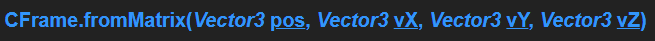# RightVector isnt working

if you copy and paste this script

``````local part = Instance.new("Part",game.Workspace)
local part2 = Instance.new("Part",game.Workspace)
part.Anchored = true
part2.Anchored = true
local pos = Vector3.new(0,0,0)
local right = Vector3.new(-1,0,0)
local top = Vector3.new(0,0,-1)
local back = Vector3.new(0,-1,0)
print(part2.CFrame)
wait(3)
part.Position = part.CFrame.RightVector * 10
part2.Position = part2.CFrame.RightVector * 10
if part.CFrame.RightVector == part2.CFrame.RightVector then
print("yes")
else
print("no")
end
``````

it prints NO for some reason
even though when i did part and part2 position = 10 studs to the right vector , it moved together!

1 Like

sigh why is that so, this is very sigh1 Like

it’s says no since you change cframe only for part2 and not part.

1 Like

yes . because want to compare the two.

1 Like

Have you tried print debugging the right vectors before the if statement?

ok, CFrame.FromMatrix() needs 4 info:pos = Position Vector (No sh*t Sherlock)
vX = Right Vector
vY = Up Vector
vZ = FowardVector (optional)

just looking to your script, you are using fowardVector like a “backVector”.
Perhaps reviewing this approach to the CFrame.FromMatrix () function can already help you understand where the error is.

oddly enough, it seems to edit part2’s rightvector to ( 1, -0, 8.74227766e-08), wich in every way shouldn’t be possible. (to my understanding of rightvectors) though this code does seem to work and do the exact same thing
`local part = Instance.new("Part") local part2 = Instance.new("Part") part.Anchored = true part2.Anchored = true part.Parent = game.Workspace part2.Parent = game.Workspace local pos = Vector3.new(0,0,0) local right = Vector3.new(-1,0,0) local top = Vector3.new(0,0,-1) local back = Vector3.new(0,-1,0) part2.CFrame = part.CFrame * CFrame.fromEulerAnglesXYZ(math.rad(90),math.rad(0),math.rad(0)) wait(3) print(part.CFrame.RightVector,"//",part2.CFrame.RightVector) if part.CFrame.RightVector == part2.CFrame.RightVector then print("yes") else print("no") end`

i made the cframe in object space, before rotating the cframe by 180 degrees, when you do part2.CFrame * CFrame.new(vector3.new(5,0,0)) it moves to the left in world space, but after rotating , the X-axis is facing our X axis which is world space, to proove that i did RightVector * 10 for both parts, which both parts moved in the same direction, despite that, it sitll prints NO even though the rightvectors are at the same place

maybe im missing something but right now what im seeing is part.CFrame.RightVector == part2.CFrame.RightVector
part.CFrame.UpVector == part2.CFrame.LookVector
part.CFrame.Z == part2.CFrame.UpVector

Well, I think vector == vector will run into floating point epsilon issues and never return true once you move away from directly assigned values, regardless of what else is going on…

2 Likes

if not, i can send an simple example to you…

1 Like

no not yet unfortunately, yes please send it to me/teach me

Ok. First Things first.
What do you wanna do exactly?
Im asking you cause we can found a better way to do.

im trying to confirm my understanding of cframe.frommatrix, what i have done is i rotated part2 cframe in a way that parts UpVector == RightVector, which is true,

when i put if part.RightVector == part2.RightVector it prints no despite the fact that when you move both the parts cframe towards the right vectors position, it moves together meaning that the rightvectors are both facing the same position

Print both of the vectors, they are probably the same except for rounding errors.